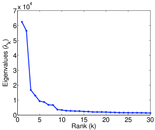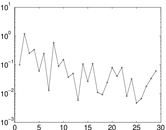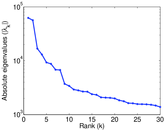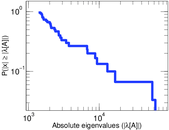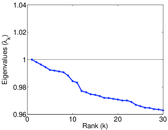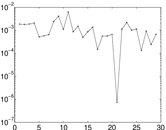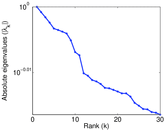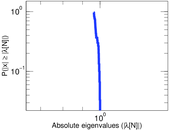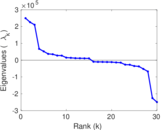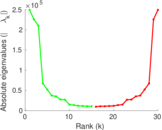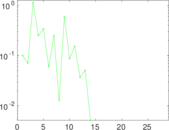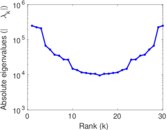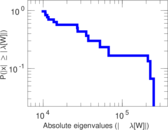# BibSonomy user–tag

This is the bipartite user–tag network from BibSonomy. Each edge represents a tag assignment. The network allows multiple edge as users may use a tag for multiple documents. The network is complete as it is taken from the official BibSonomy dump.

 Code `But` Internal name `bibsonomy-2ut` Name BibSonomy user–tag Data source http://www.kde.cs.uni-kassel.de/bibsonomy/dumps AvailabilityDataset is available for download Consistency checkDataset passed all tests Category Interaction network Dataset timestamp 2010 Node meaning User, tag Edge meaning Assignment Network formatBipartite, undirected Edge typeUnweighted, multiple edges Temporal dataEdges are annotated with timestamps

## Statistics

 Size n = 210,467 Left size n1 = 5,794 Right size n2 = 204,673 Volume m = 2,555,080 Unique edge count m̿ = 453,987 Wedge count s = 991,843,705 Claw count z = 4,596,638,282,952 Cross count x = 19,818,609,712,155,388 Square count q = 305,062,057 4-Tour count T4 = 6,409,052,574 Maximum degree dmax = 428,436 Maximum left degree d1max = 428,436 Maximum right degree d2max = 182,908 Average degree d = 24.280 1 Average left degree d1 = 440.987 Average right degree d2 = 12.483 7 Fill p = 0.000 382 829 Average edge multiplicity m̃ = 5.628 09 Size of LCC N = 209,357 Diameter δ = 12 50-Percentile effective diameter δ0.5 = 3.517 73 90-Percentile effective diameter δ0.9 = 4.011 15 Median distance δM = 4 Mean distance δm = 4.051 36 Gini coefficient G = 0.926 128 Balanced inequality ratio P = 0.089 785 8 Left balanced inequality ratio P1 = 0.080 483 2 Right balanced inequality ratio P2 = 0.134 076 Relative edge distribution entropy Her = 0.772 367 Power law exponent γ = 3.674 91 Tail power law exponent γt = 1.961 00 Tail power law exponent with p γ3 = 1.961 00 p-value p = 0.271 000 Left tail power law exponent with p γ3,1 = 1.681 00 Left p-value p1 = 0.000 00 Right tail power law exponent with p γ3,2 = 2.201 00 Right p-value p2 = 0.000 00 Spectral norm α = 62,391.4 Algebraic connectivity a = 0.016 601 9 Spectral separation |λ1[A] / λ2[A]| = 1.105 36 Controllability C = 199,969 Relative controllability Cr = 0.950 120

## Plots

### Degree distribution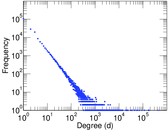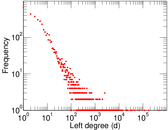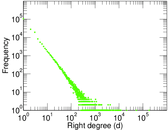### Cumulative degree distribution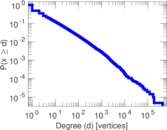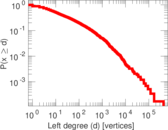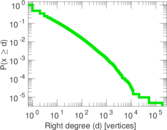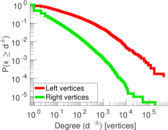### Lorenz curve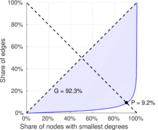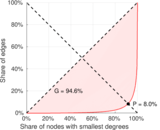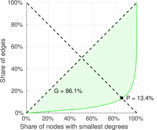### Spectral distribution of the adjacency matrix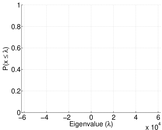### Spectral distribution of the normalized adjacency matrix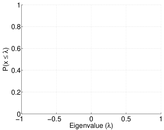### Spectral distribution of the Laplacian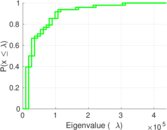### Spectral graph drawing based on the adjacency matrix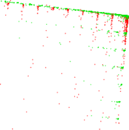### Spectral graph drawing based on the Laplacian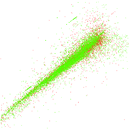### Spectral graph drawing based on the normalized adjacency matrix### Degree assortativity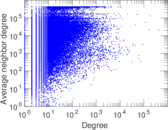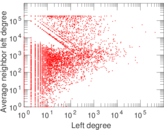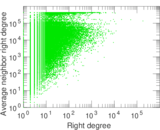### Zipf plot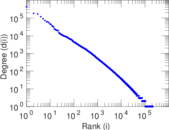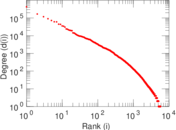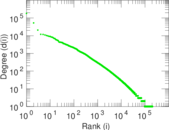### Hop distribution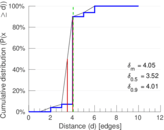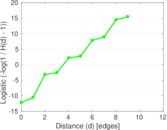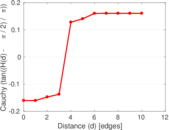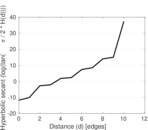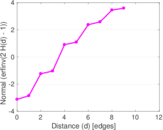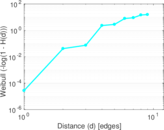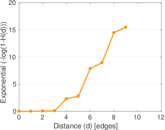### Delaunay graph drawing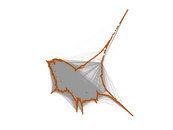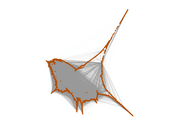### Edge weight/multiplicity distribution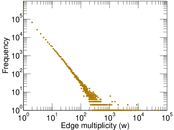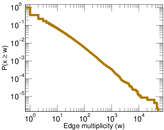### Temporal distribution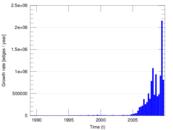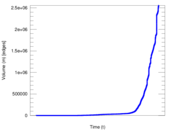### Diameter/density evolution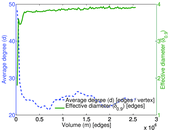### Inter-event distribution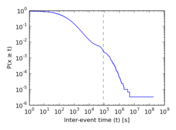### Node-level inter-event distribution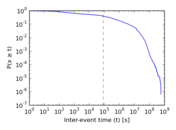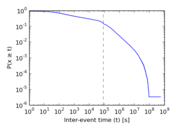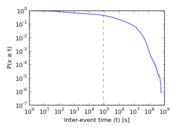### Matrix decompositions plots